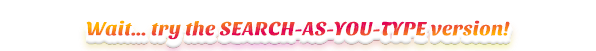# Lambdas

A lambda function is a small anonymous function. A lambda function can take any number of arguments, but can only have one expression.

## Lambda function syntax

lambda arguments: expression


## Lambda function to add 3 numbers

# lambda takes 3 arguments
add_lambda = lambda x, y, z: x + y + z
6


## Lambda passed as argument to function sorted

# list of tuples
lst = [(13, 'a'), (4, 'd'), (9, 'b'), (1, 'e')]
# sort by second item of the tuple
lst_sort = sorted(lst, key=lambda x:x)

>>> lst_sort
[(13, 'a'), (9, 'b'), (4, 'd'), (1, 'e')]


## Lambda passed as argument to function sort

# list of tuples
lst = [(13, 'a'), (4, 'd'), (9, 'b'), (1, 'e')]
# sort (in place) by second item of the tuple
lst.sort(key=lambda x:x)

>>> lst
[(13, 'a'), (9, 'b'), (4, 'd'), (1, 'e')]


## Lambda passed as argument to function max

lst = ['1', '100', '111', '2']

# strings are compared lexicographically
max_lex = max(lst)

>>> max_lex
'2'

# use lambda to convert strings to numeric before applying max
max_num = max(lst, key=lambda x: int(x))

>>> max_num
'111'